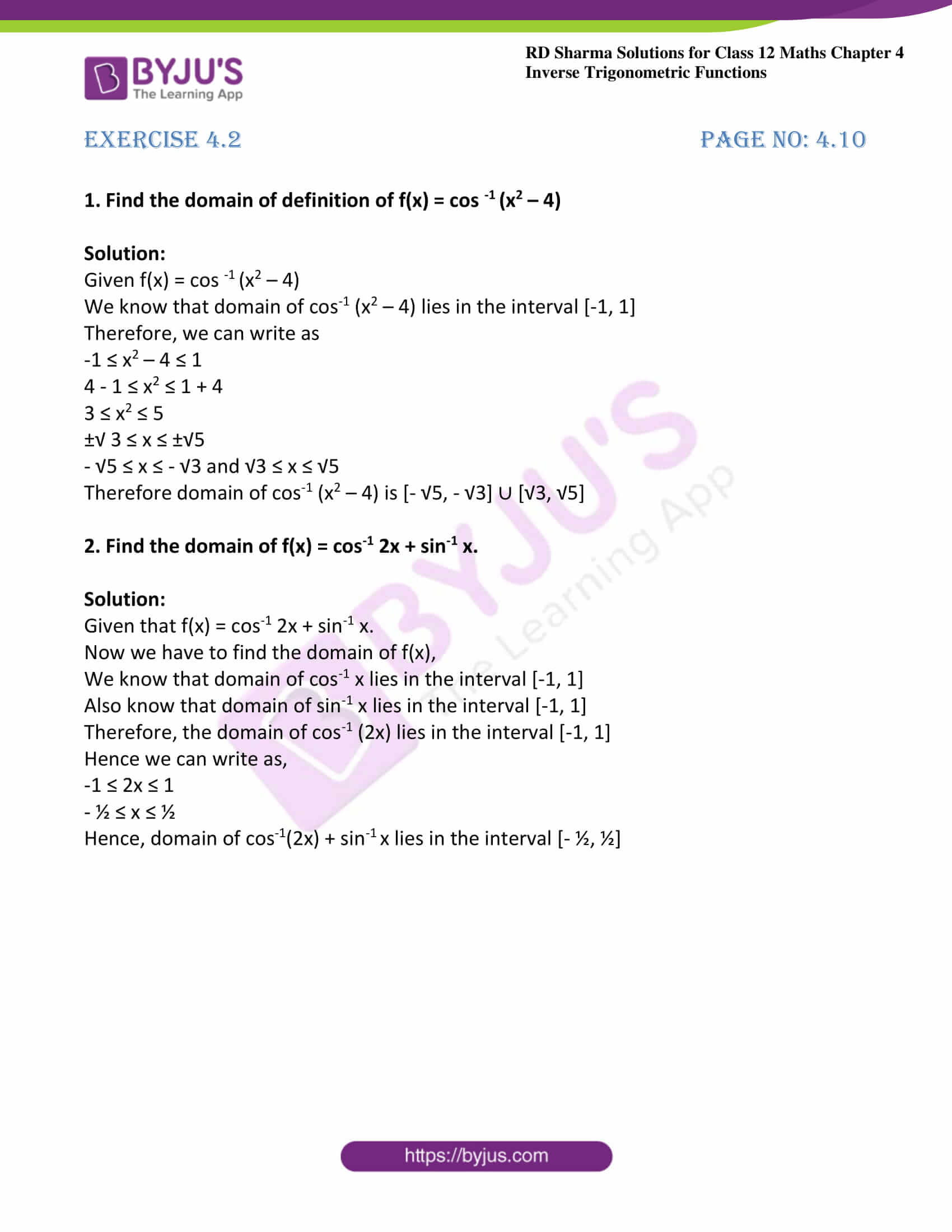# RD Sharma Solutions For Class 12 Maths Exercise 4.2 Chapter 4 Inverse Trigonometric Functions

RD Sharma Solutions Class 12 Maths Exercise 4.2 Chapter 4 Inverse Trigonometric Functions is provided here. This exercise covers the problems based on the inverse of cosine functions. These problems are solved in the best possible way to improve logical thinking skills among students. The faculty has vast experience in respective field design solutions relevant to the exam pattern.

The RD Sharma Solutions contain explanations in a comprehensive manner to make it interesting for the students while solving. To score good marks in the exam, students can refer RD Sharma Solutions Class 12 Maths Chapter 4 Inverse Trigonometric Functions Exercise 4.2, which are available here.

## Download the PDF of RD Sharma Solutions For Class 12 Chapter 4 – Inverse Trigonometric Functions Exercise 4.2### Exercise 4.2 Page No: 4.10

1. Find the domain of definition of f(x) = cos -1 (x2 – 4)

Solution:

Given f(x) = cos -1 (x2 – 4)

We know that domain of cos-1 (x2 – 4) lies in the interval [-1, 1]

Therefore, we can write as

-1 ≤ x2 – 4 ≤ 1

4 – 1 ≤ x2 ≤ 1 + 4

3 ≤ x2 ≤ 5

±√ 3 ≤ x ≤ ±√5

– √5 ≤ x ≤ – √3 and √3 ≤ x ≤ √5

Therefore domain of cos-1 (x2 – 4) is [- √5, – √3] ∪ [√3, √5]

2. Find the domain of f(x) = cos-1 2x + sin-1 x.

Solution:

Given that f(x) = cos-1 2x + sin-1 x.

Now we have to find the domain of f(x),

We know that domain of cos-1 x lies in the interval [-1, 1]

Also know that domain of sin-1 x lies in the interval [-1, 1]

Therefore, the domain of cos-1 (2x) lies in the interval [-1, 1]

Hence we can write as,

-1 ≤ 2x ≤ 1

– ½ ≤ x ≤ ½

Hence, domain of cos-1(2x) + sin-1 x lies in the interval [- ½, ½]

### Access other exercises of RD Sharma Solutions For Class 12 Chapter 4 – Inverse Trigonometric Functions

Exercise 4.1 Solutions

Exercise 4.3 Solutions

Exercise 4.4 Solutions

Exercise 4.5 Solutions

Exercise 4.6 Solutions

Exercise 4.7 Solutions

Exercise 4.8 Solutions

Exercise 4.9 Solutions

Exercise 4.10 Solutions

Exercise 4.11 Solutions

Exercise 4.12 Solutions

Exercise 4.13 Solutions

Exercise 4.14 Solutions